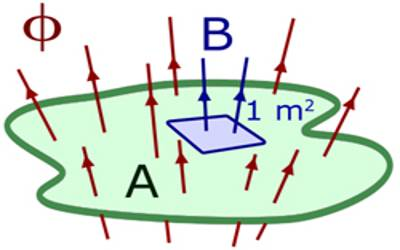# Flux Density

Flux density is the amount of magnetic, electric, or other flux passing through a unit area. If a plane or a coil (real or fictitious) is placed in a magnetic field perpendicular to the magnetic field lines, then the total number of field lines passing through the unit area of that plane or coil is called flux density in that plane.

The number of magnetic field lines or magnetic flux incident normally on the unit area of a real or fictitious plane is called flux density in that plane. It is also called magnetic field or magnetic induction. Magnetic flux density is defined as the force acting per unit current per unit length on a wire placed at right angles to the magnetic field.Unit of the magnetic field or flux density:

In S.I. the method the unit of flux density or magnetic field is,

B = φ/A = Weber/meter2 (Wbm-2). It is called Tesla. Tesla is denoted by T.

By putting the units of the quantities on right hand side of the equation (direction of magnetic field), we get the unit of B,

B = F / qv = N / Cms-1 = N / Cs-1m = N/Am = NA-1m-1.

So, 1 Tesla = 1 Wbm-2 = 1 NA-1m-1 = 104 gauss.

The definition of 1 Tesla is given below:

1 Testa: If 1 coulomb of charge is in the motion perpendicular to the direction of magnetic field with a velocity of 1 ms-1 and experiences a force of 1N, then the Magnitude of that magnetic field is called 1 Testa (T).

Testa is a very large unit. In many cases, the magnitude of the magnetic field is much less than l Testa. For example, the magnetic field on the surface of the earth is approximately l0-4 T. So, in some cases Gauss (G) is used as a convenient unit.

l Gauss = 10-4 T.